# Comparing fractions

Here you’ll learn about comparing fractions, including how to compare fractions with different denominators to decide which of two fractions is the smaller fraction and which is the larger fraction

Students will first learn about comparing fractions as part of numbers and operations in fractions in 4th grade.

## What is comparing fractions?

Comparing fractions is deciding whether one fraction is larger than, smaller than or equal to another.

To do this you can use common denominators, common numerators or compare to benchmark fractions. Use inequality symbols < (less than) and > (greater than) to write the comparison.

For example, which fraction is larger,

### Common denominators

The denominators are not the same, so you can create equivalent fractions. Multiply each fraction by the opposite denominator.

\cfrac{24}{40} has 24 parts shaded in and \cfrac{15}{40} has 15 parts shaded in.

Since the parts are the same size, \cfrac{24}{40} is larger.

So, \cfrac{3}{5} is larger than \cfrac{3}{8}.

You write this as \cfrac{3}{5}>\cfrac{3}{8}.

### Common numerators

\cfrac{3}{5} and \cfrac{3}{8} have the same numerators, which means they have the same number of parts.

You can use the denominator to decide which parts are larger. Smaller denominators means a larger part of a whole. Larger denominators means a smaller part of a whole.

Fifths are larger, so 3 fifths will be larger than 3 eighths.

So, \cfrac{3}{5} is larger than \cfrac{3}{8}.

You can write this as \cfrac{3}{5}>\cfrac{3}{8}.

### Benchmark fractions

You can also compare fractions by using the benchmarks 0, \cfrac{1}{2} and 1.

\cfrac{3}{5} \rightarrow 3 is more than half of 5, so \cfrac{3}{5} is greater than \cfrac{1}{2}.

\cfrac{3}{8} \rightarrow 3 is less than half of 8, so \cfrac{3}{8} is less than \cfrac{1}{2}.

So, \cfrac{3}{5} is larger than \cfrac{3}{8}.

You can write this as \cfrac{3}{5}>\cfrac{3}{8}.

### What is comparing fractions?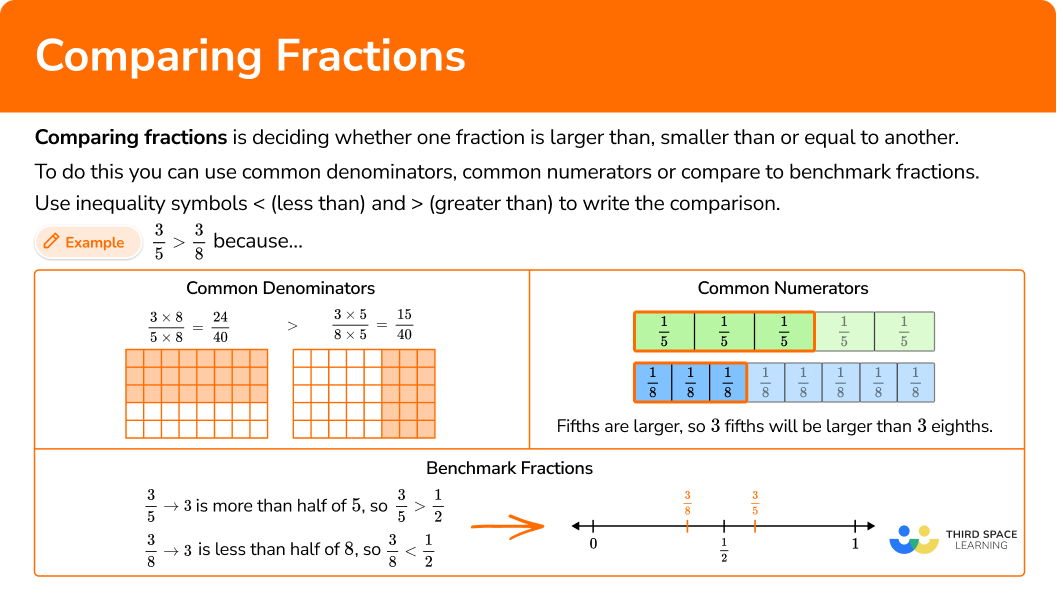## Common Core State Standards

How does this relate to 4th grade math?

• Grade 4: Numbers and Operations – Fractions (4.NF.A.2)
Compare two fractions with different numerators and different denominators, e.g., by creating common denominators or numerators, or by comparing to a benchmark fraction such as \cfrac{1}{2}. Recognize that comparisons are valid only when the two fractions refer to the same whole. Record the results of comparisons with symbols > , =, or < , and justify the conclusions, e.g., by using a visual fraction model.

## How to compare fractions

In order to compare fractions using common denominators:

1. See if the fractions have like denominators.
2. Make equivalent fractions if needed.
3. Write the answer using the original fractions.

In order to compare fractions using common numerators:

1. See if fractions have like numerators.
2. Make equivalent fractions if needed.
3. Write the answer using the original fractions.

In order to compare fractions using benchmark fractions:

1. Decide how the fraction compares to \bf{0} , \bf{\cfrac{1}{2}} and \bf{1} .
2. Write the answer using the original fractions.

## Comparing fractions examples

### Example 1: compare using common denominators

Compare: \cfrac{2}{5} \bigcirc \cfrac{3}{4}.

1. See if the fractions have like denominators.

The fractions do not have the same denominators (bottom numbers).

2Make equivalent fractions if needed.

To create a common denominator, multiply each fraction by the opposite denominator.

\hspace{0.5cm} \cfrac{2}{5}=\cfrac{2 \; \times \; 4}{5 \; \times \; 4}=\cfrac{8}{20} \hspace{0.5cm} and \hspace{0.5cm} \cfrac{3}{4}=\cfrac{3 \; \times \; 5}{4 \; \times \; 5}=\cfrac{15}{20}

3Write the answer using the original fractions.

\cfrac{8}{20} has 8 parts shaded in and \cfrac{15}{20} has 15 parts shaded in.

Since the parts are the same size, \cfrac{8}{20} is smaller.

So, \cfrac{2}{5} is smaller than \cfrac{3}{4}.

You write this as \cfrac{2}{5} < \cfrac{3}{4}.

You could have also used benchmark fractions to compare these fractions.

\cfrac{2}{5} is less than \cfrac{1}{2} and \cfrac{3}{4} is greater than \cfrac{1}{2}.

### Example 2: compare using common denominators

Compare: \cfrac{4}{6} \bigcirc \cfrac{8}{12}.

The fractions do not have the same denominators (bottom numbers).

To create a common denominator, multiply each fraction by the opposite denominator.

\hspace{0.2cm} \cfrac{4}{6}=\cfrac{4 \; \times \; 12}{6 \; \times \; 12}=\cfrac{48}{72} \hspace{0.5cm} and \hspace{0.5cm} \cfrac{8}{12}=\cfrac{8 \; \times \; 6}{12 \; \times \; 6}=\cfrac{48}{72}

(You can also create common denominators by doubling \cfrac{4}{6}=\cfrac{4 \; \times \; 2}{6 \; \times \; 2}=\cfrac{8}{12}.)

Both fractions \cfrac{48}{72} have 48 parts shaded.

Since the parts are the same size, the fractions are equal.

So, \cfrac{4}{6} and \cfrac{8}{12} are equal.

You write this as \cfrac{4}{6}=\cfrac{8}{12}.

### Example 3: compare using common numerators

Compare: \cfrac{7}{8} \bigcirc \cfrac{7}{10}.

The fractions have the same numerators (top numbers).

You do not need to use equivalent fractions, you can compare with the common numerators.

Eighths are larger, so 7 eighths will be larger than 7 tenths.

So, \cfrac{7}{8} is larger than \cfrac{7}{10}.

You write this as \cfrac{7}{8}>\cfrac{7}{10}.

### Example 4: compare using common numerators

Compare: \cfrac{2}{10} \bigcirc \cfrac{1}{12}.

The fractions do not have the same numerators (top numbers).

To create a common numerator, multiply each fraction by the opposite numerator.

\cfrac{2}{10}=\cfrac{2 \; \times \; 1}{10 \; \times \; 1}=\cfrac{2}{10} \hspace{0.5cm} and \hspace{0.5cm} \cfrac{1}{12}=\cfrac{1 \; \times \; 2}{12 \; \times \; 2}=\cfrac{2}{24}

Tenths are larger, so 2 tenths will be larger than 2 twenty-fourths.

So, \cfrac{2}{10} is larger than \cfrac{2}{24}.

You write this as \cfrac{2}{10}>\cfrac{1}{12}.

### Example 5: compare using benchmark fractions

Compare: \cfrac{3}{6} \bigcirc \cfrac{4}{5}.

\cfrac{3}{6} is equal to \cfrac{1}{2} and \cfrac{4}{5} is greater than \cfrac{1}{2}.

So, \cfrac{3}{6} is smaller than \cfrac{4}{5}.

You write this as \cfrac{3}{6}<\cfrac{4}{5}.

### Example 6: compare using benchmark fractions

Compare: \cfrac{1}{4} \bigcirc \cfrac{2}{3}.

\cfrac{1}{4} is less than \cfrac{1}{2} and \cfrac{2}{3} is greater than \cfrac{1}{2}.

So, \cfrac{1}{4} is smaller than \cfrac{2}{3}.

You write this as \cfrac{1}{4}<\cfrac{2}{3}.

### Teaching tips for comparing fractions

• Instead of telling students which method to use, give students opportunities to try each method when comparing different pairs of fractions. This helps them not only remember the methods, but grow ideas about which methods work best for different fraction comparisons.

• Fraction work in 3rd grade centers around understanding through models; particularly area models and number lines. To build on this in 4th grade, always have physical or digital models available for students to use when necessary.

• Avoid teaching students to cross multiply. Students are not yet ready to understand the math behind cross multiplication. The purpose of comparing fractions at this level is for students to continue making sense of fraction sizes. Students should be thinking deeply about the process used to compare, not memorizing a rote procedure.

### Our favorite mistakes

• Mixing up the inequality symbols
Make sure you get them the right way around, < is “less than” and > is “greater than”. An easy way to remember them is to think of these images of blocks.

• Thinking using fractions is only one way to compare fractions
In some cases, it may be easier to use decimals instead. For example, comparing \cfrac{1}{2} and \cfrac{3}{10}. These fractions as decimals would be 0.5 and 0.3.
Therefore \cfrac{1}{2} is the smallest fraction and \cfrac{3}{10} is the larger fraction.

### Practice comparing fractions questions

1. Compare: \cfrac{5}{8} \bigcirc \cfrac{4}{10}.

\cfrac{5}{8}>\cfrac{4}{10}\cfrac{5}{8}<\cfrac{4}{10}\cfrac{5}{8}=\cfrac{4}{10}\cfrac{5}{8}\le\cfrac{4}{10}\cfrac{5}{8} is greater than \cfrac{1}{2} and \cfrac{4}{10} is less than than \cfrac{1}{2}.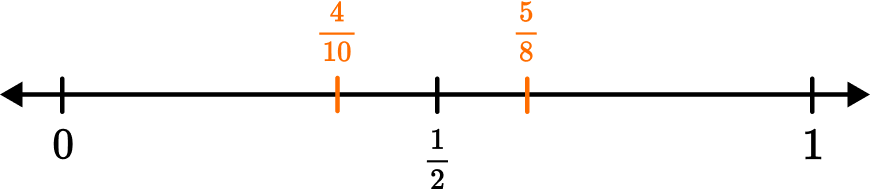So, \cfrac{5}{8} is greater than \cfrac{4}{10}.

You write this as \cfrac{5}{8}>\cfrac{4}{10}.

2. Compare: \cfrac{6}{8} \bigcirc \cfrac{5}{6}.

\cfrac{6}{8}=\cfrac{5}{6}\cfrac{6}{8}\ge\cfrac{5}{6}\cfrac{6}{8}>\cfrac{5}{6}\cfrac{6}{8}<\cfrac{5}{6}The denominators are not like.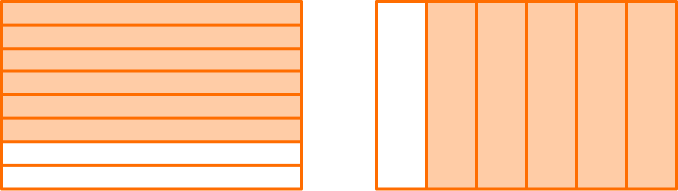To create a common denominator, multiply each fraction by the opposite denominator.

\hspace{0.5cm} \cfrac{6}{8}=\cfrac{6 \; \times \; 6}{8 \; \times \; 6}=\cfrac{36}{48} \hspace{0.5cm} and \hspace{0.5cm} \cfrac{5}{6}=\cfrac{5 \; \times \; 8}{6 \; \times \; 8}=\cfrac{40}{48}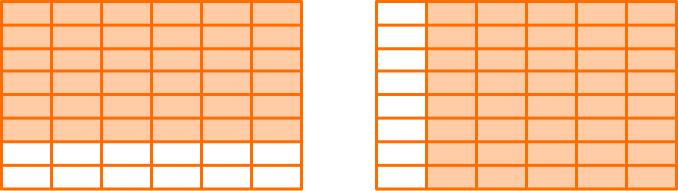\cfrac{36}{48} has 36 parts shaded in and \cfrac{40}{48} has 40 parts shaded in.

Since the parts are the same size, \cfrac{36}{48} is smaller.

So, \cfrac{6}{8} is smaller than \cfrac{5}{6}.

You write this as \cfrac{6}{8}<\cfrac{5}{6}.

3. Compare: \cfrac{3}{10} \bigcirc \cfrac{3}{12}.

\cfrac{3}{10}<\cfrac{3}{12}\cfrac{3}{10}>\cfrac{3}{12}\cfrac{3}{10}=\cfrac{3}{12}\cfrac{3}{10}\le\cfrac{3}{12}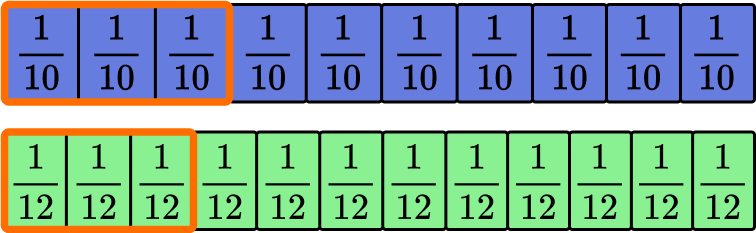Tenths are larger, so 3 tenths will be larger than 3 twelfths.

You write this as \cfrac{3}{10}>\cfrac{3}{12}.

4. Compare: \cfrac{5}{6} \bigcirc \cfrac{10}{12}.

\cfrac{5}{6}>\cfrac{10}{12}\cfrac{5}{6}\cfrac{10}{12}\cfrac{5}{6}<\cfrac{10}{12}\cfrac{5}{6}=\cfrac{10}{12}The denominators are not like.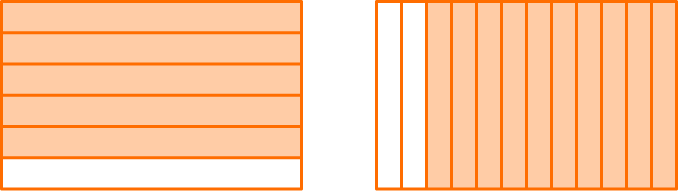To create a common denominator, multiply each fraction by the opposite denominator.

\hspace{0.2cm} \cfrac{5}{6}=\cfrac{5 \; \times \; 12}{6 \; \times \; 12}=\cfrac{60}{72} \hspace{0.5cm} and \hspace{0.5cm} \cfrac{10}{12}=\cfrac{10 \; \times \; 6}{12 \; \times \; 6}=\cfrac{60}{72}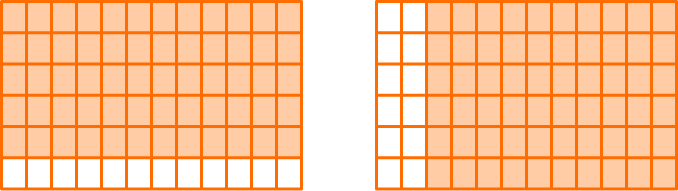(You can also create common denominators by doubling \cfrac{5}{6}=\cfrac{5 \; \times \; 2}{6 \; \times \; 2}=\cfrac{10}{12}.)

Both fractions \cfrac{60}{72} have 60 parts shaded.

Since the parts are the same size, the fractions are equal.

So, \cfrac{5}{6} and \cfrac{10}{12} are equal.

You write this as \cfrac{5}{6}=\cfrac{10}{12}.

5. Which of the following fractions is the smallest?
\cfrac{2}{3} \hspace{0.5cm} \cfrac{5}{6} \hspace{0.5cm} \cfrac{3}{4} \hspace{0.5cm} \cfrac{11}{12}

\cfrac{5}{6}\cfrac{11}{12}\cfrac{3}{4}\cfrac{2}{3}Each fraction is one part away from a whole.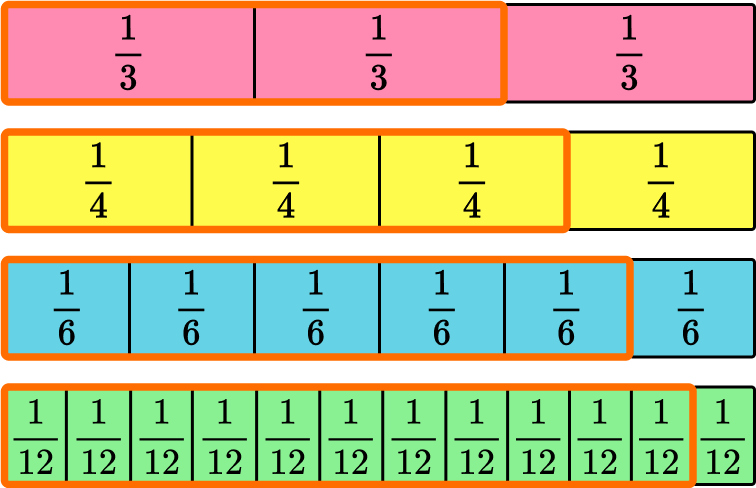Since thirds are the largest parts, \cfrac{2}{3} is the farthest from 1 whole and is the smallest.

You can also solve by finding common denominators. All the fractions have a multiple of 12.

\cfrac{2 \; \times \; 4}{3 \; \times \; 4}=\cfrac{8}{12} \hspace{0.5cm} \cfrac{5 \; \times \; 2}{6 \; \times \; 2}=\cfrac{10}{12} \hspace{0.5cm} \cfrac{3 \; \times \; 3}{4 \; \times \; 3}=\cfrac{9}{12} \hspace{0.5cm} \cfrac{11}{12}

Now compare the numerators. 8 is the smallest numerator, so \cfrac{2}{3} is the smallest fraction.

6. Which fraction is the largest?
\cfrac{7}{10} \hspace{0.5cm} \cfrac{4}{5} \hspace{0.5cm} \cfrac{1}{2} \hspace{0.5cm} \cfrac{3}{8}

\cfrac{7}{10}\cfrac{4}{5}\cfrac{1}{2}\cfrac{3}{8}\cfrac{3}{8} is less than \cfrac{1}{2}.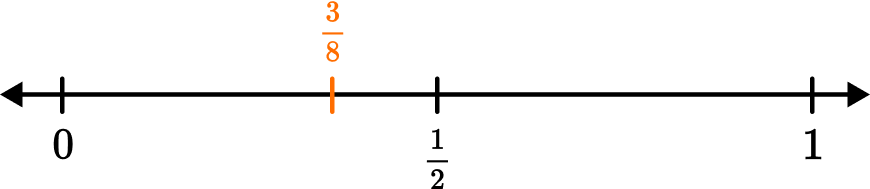\cfrac{7}{10} and \cfrac{4}{5} are greater than \cfrac{1}{2}, so one of those will be the largest.

You can use a common denominator to compare \cfrac{7}{10} and \cfrac{4}{5}.

\cfrac{7 \; \times \; 5}{10 \; \times \; 5}=\cfrac{35}{50} \hspace{0.5cm} and \hspace{0.5cm} \cfrac{4 \; \times \; 10}{5 \; \times \; 10}=\cfrac{40}{50}

(You can also create common denominators by doubling \cfrac{4}{5}=\cfrac{4 \; \times \; 2}{5 \; \times \; 2}=\cfrac{8}{10}.)

Now that the denominators are the same, the parts are the same size.

\cfrac{40}{50} has a larger numerator than \cfrac{35}{50}, which means it has more parts.

So, \cfrac{4}{5} is the largest.

## Comparing fractions FAQs

Do you have to use the least common denominator when comparing?

No, you can use any factor to create a common denominator to compare fractions.

Can you compare other types of fractions, like mixed numbers and improper?

Yes, the same strategies shown above can be used for improper fractions and mixed numbers. There is an additional step for mixed numbers. You would either need to compare the whole numbers first, before the fraction or convert the mixed number to an improper fraction before comparing.

Can you compare more than two fractions?

Yes, you can compare a set of fractions by ordering them from least to greatest or greatest to least. They can be listed or written as an inequality comparison.

## Still stuck?

At Third Space Learning, we specialize in helping teachers and school leaders to provide personalized math support for more of their students through high-quality, online one-on-one math tutoring delivered by subject experts.

Each week, our tutors support thousands of students who are at risk of not meeting their grade-level expectations, and help accelerate their progress and boost their confidence.